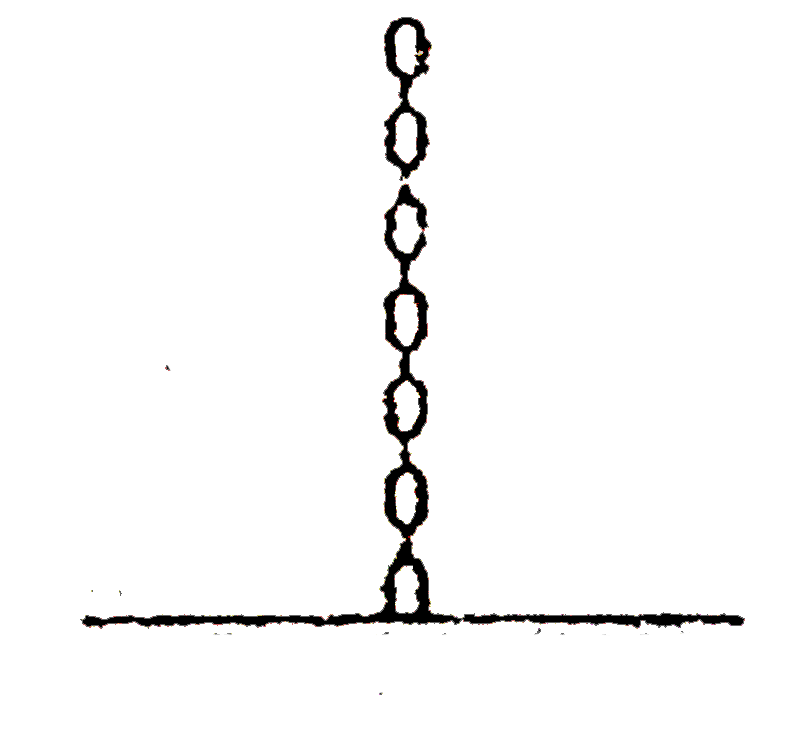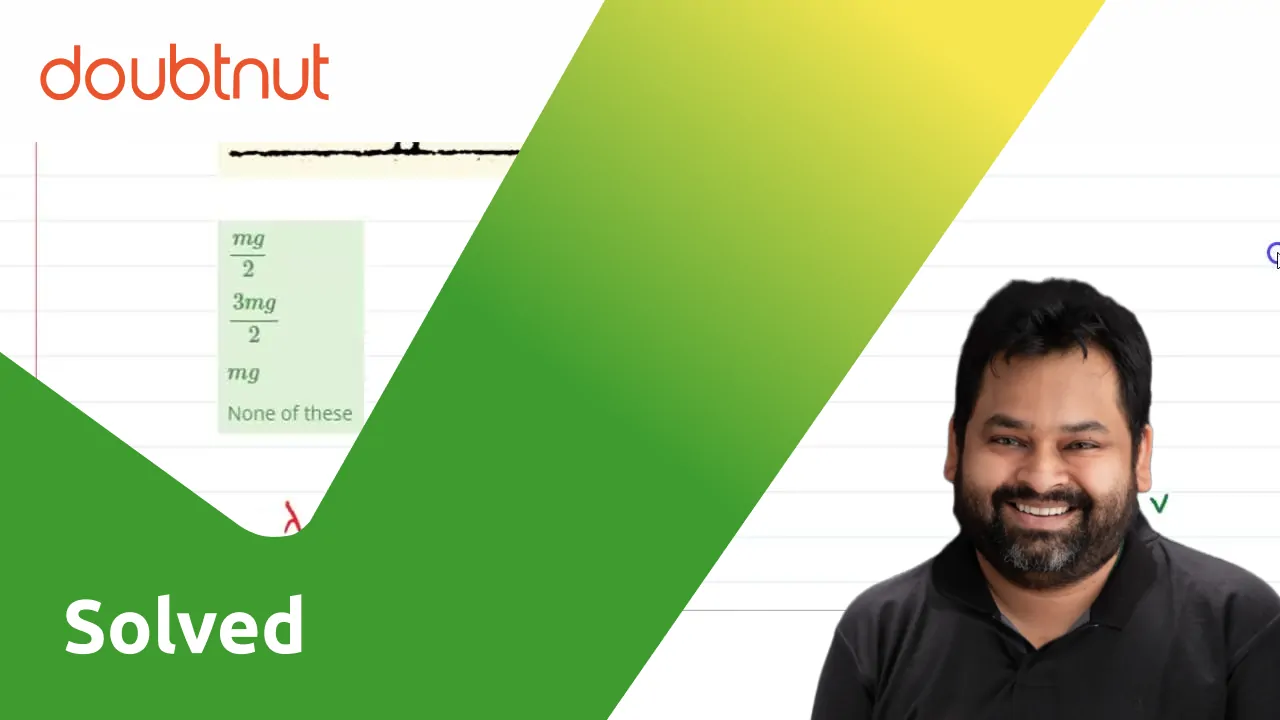Doubtnut App - Get 50 Lakh+ video solutions better than GoogleInstall Now

# A uniform chain of mass m and length l hangs on a thread and touches the surface of a table by its lower end. Thread breaks suddenly. Find the force exerted by the table on the chain when half of its length has fallen on the table. The fallen part does not form heapSolution in Gujrati

A

mg2

B

3mg2

C

mg

D

None of these

Text Solution
Verified by Experts

## Ans.(2) At any instant of time let velocity of part of chain which is just coming in contact with surface =v and dx= length of chain reaches on surface in time dt then momentum carried by this part dp=(dm)v ∴ rate of change of momentum of chain dpdt=dmdtv=λdxdtv=λv2=(ml)v2 as v=√2g(l2)=√gl so dpdt=ml(gl)=mg weight of part on the surface =mg2 ∴ net force by chain on surface =mg+mg2=32mg